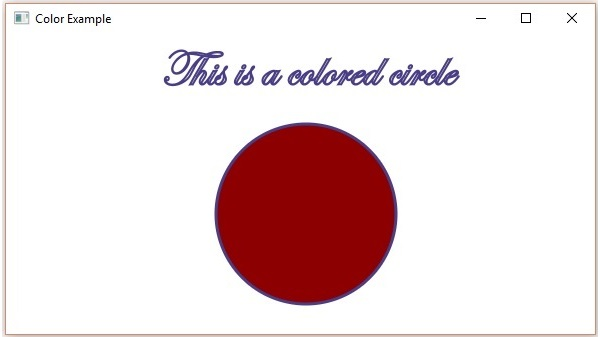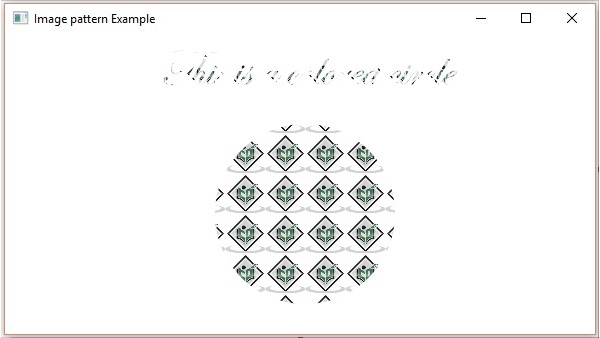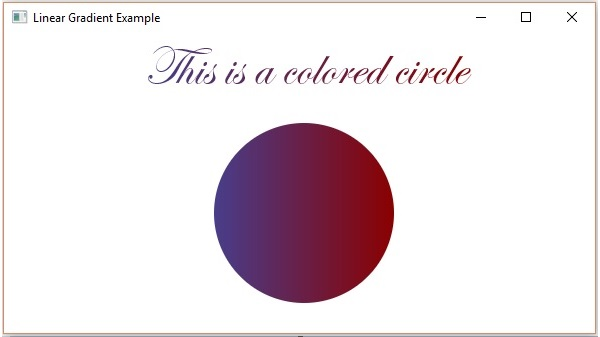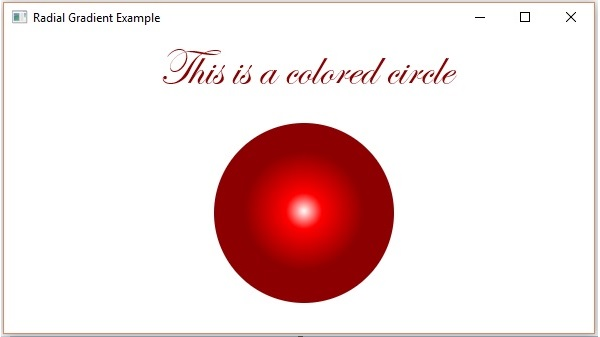# JavaFX - Colors

To apply colors to an application, JavaFX provides various classes in the package javafx.scene.paint package. This package contains an abstract class named Paint and it is the base class of all the classes that are used to apply colors.

Using these classes, you can apply colors in the following patterns −

• Uniform − In this pattern, color is applied uniformly throughout node.

• Image Pattern − This lets you to fill the region of the node with an image pattern.

• Gradient − In this pattern, the color applied to the node varies from one point to the other. It has two kinds of gradients namely Linear Gradient and Radial Gradient.

All those node classes to which you can apply color such as Shape, Text (including Scene), have methods named setFill() and setStroke(). These will help to set the color values of the nodes and their strokes respectively.

These methods accept an object of type Paint. Therefore, to create either of these type of images, you need to instantiate these classes and pass the object as a parameter to these methods.

## Applying Color to the Nodes

To set uniform color pattern to the nodes, you need to pass an object of the class color to the setFill(), setStroke() methods as follows −

```//Setting color to the text
Color color = new Color.BEIGE
text.setFill(color);

//Setting color to the stroke
Color color = new Color.DARKSLATEBLUE
circle.setStroke(color);
```

In the above code block, we are using the static variables of the color class to create a color object.

In the same way, you can also use the RGB values or HSB standard of coloring or web hash codes of colors as shown below −

```//creating color object by passing RGB values
Color c = Color.rgb(0,0,255);

//creating color object by passing HSB values
Color c = Color.hsb(270,1.0,1.0);

//creating color object by passing the hash code for web
Color c = Color.web("0x0000FF",1.0);
```

### Example

Following is an example which demonstrates, how to apply color to the nodes in JavaFX. Here, we are creating a circle and text nodes and applying colors to them.

Save this code in a file with the name ColorExample.java.

```import javafx.application.Application;
import javafx.scene.Group;
import javafx.scene.Scene;
import javafx.scene.paint.Color;
import javafx.stage.Stage;
import javafx.scene.shape.Circle;
import javafx.scene.text.Font;
import javafx.scene.text.Text;

public class ColorExample extends Application {
@Override
public void start(Stage stage) {
//Drawing a Circle
Circle circle = new Circle();

//Setting the properties of the circle
circle.setCenterX(300.0f);
circle.setCenterY(180.0f);

//Setting color to the circle
circle.setFill(Color.DARKRED);

//Setting the stroke width
circle.setStrokeWidth(3);

//Setting color to the stroke
circle.setStroke(Color.DARKSLATEBLUE);

//Drawing a text
Text text = new Text("This is a colored circle");

//Setting the font of the text
text.setFont(Font.font("Edwardian Script ITC", 50));

//Setting the position of the text
text.setX(155);
text.setY(50);

//Setting color to the text
text.setFill(Color.BEIGE);
text.setStrokeWidth(2);
text.setStroke(Color.DARKSLATEBLUE);

//Creating a Group object
Group root = new Group(circle, text);

//Creating a scene object
Scene scene = new Scene(root, 600, 300);

//Setting title to the Stage
stage.setTitle("Color Example");

stage.setScene(scene);

//Displaying the contents of the stage
stage.show();
}
public static void main(String args[]){
launch(args);
}
}
```

Compile and execute the saved java file from the command prompt using the following commands.

```Javac ColorExample.java
java ColorExample
```

On executing, the above program generates a JavaFX window as follows −## Applying Image Pattern to the Nodes

To apply an image pattern to the nodes, instantiate the ImagePattern class and pass its object to the setFill(), setStroke() methods.

The constructor of this class accepts six parameters namely −

• Image − The object of the image using which you want to create the pattern.

• x and y − Double variables representing the (x, y) coordinates of origin of the anchor rectangle.

• height and width − Double variables representing the height and width of the image that is used to create a pattern.

• isProportional − This is a Boolean Variable; on setting this property to true, the start and end locations are set to be proportional.

```ImagePattern radialGradient = new ImagePattern(dots, 20, 20, 40, 40, false);
```

### Example

Following is an example which demonstrates how to apply image pattern to the nodes in JavaFX. Here, we are creating a circle and a text node and applying an image pattern to them.

Save this code in a file with name ImagePatternExample.java.

```import javafx.application.Application;
import javafx.scene.Group;
import javafx.scene.Scene;
import javafx.scene.image.Image;

import javafx.scene.paint.Color;
import javafx.scene.paint.ImagePattern;
import javafx.scene.paint.Stop;

import javafx.stage.Stage;
import javafx.scene.shape.Circle;
import javafx.scene.text.Font;
import javafx.scene.text.Text;

public class ImagePatternExample extends Application {
@Override
public void start(Stage stage) {
//Drawing a Circle
Circle circle = new Circle();

//Setting the properties of the circle
circle.setCenterX(300.0f);
circle.setCenterY(180.0f);

//Drawing a text
Text text = new Text("This is a colored circle");

//Setting the font of the text
text.setFont(Font.font("Edwardian Script ITC", 50));

//Setting the position of the text
text.setX(155);
text.setY(50);

//Setting the image pattern
+ "/images?q=tbn:ANd9GcRQub4GvEezKMsiIf67U"

//Setting the linear gradient to the circle and text

//Creating a Group object
Group root = new Group(circle, text);

//Creating a scene object
Scene scene = new Scene(root, 600, 300);

//Setting title to the Stage
stage.setTitle("Image pattern Example");

stage.setScene(scene);

//Displaying the contents of the stage
stage.show();
}
public static void main(String args[]){
launch(args);
}
}
```

Compile and execute the saved java file from the command prompt using the following commands.

```Javac ImagePatternExample.java
java ImagePatternExample
```

On executing, the above program generates a JavaFX window as follows −To apply a Linear Gradient Pattern to the nodes, instantiate the LinearGradient class and pass its object to the setFill(), setStroke() methods.

The constructor of this class accepts five parameters namely −

• startX, startY − These double properties represent the x and y coordinates of the starting point of the gradient.

• endX, endY − These double properties represent the x and y coordinates of the ending point of the gradient.

• cycleMethod − This argument defines how the regions outside the color gradient bounds, defined by the starting and ending points, should be filled.

• proportional − This is a Boolean Variable; on setting this property to true, the start and end locations are set to a proportion.

• Stops − This argument defines the color-stop points along the gradient line.

```//Setting the linear gradient
Stop[] stops = new Stop[] {
new Stop(0, Color.DARKSLATEBLUE),
new Stop(1, Color.DARKRED)
};
new LinearGradient(0, 0, 1, 0, true, CycleMethod.NO_CYCLE, stops);
```

### Example

Following is an example which demonstrates how to apply a gradient pattern to the nodes in JavaFX. Here, we are creating a circle and a text nodes and applying linear gradient pattern to them.

Save this code in a file with name LinearGradientExample.java.

```import javafx.application.Application;
import javafx.scene.Group;
import javafx.scene.Scene;

import javafx.scene.paint.Color;
import javafx.scene.paint.CycleMethod;
import javafx.scene.paint.Stop;

import javafx.stage.Stage;
import javafx.scene.shape.Circle;
import javafx.scene.text.Font;
import javafx.scene.text.Text;

public class LinearGradientExample extends Application {
@Override
public void start(Stage stage) {
//Drawing a Circle
Circle circle = new Circle();

//Setting the properties of the circle
circle.setCenterX(300.0f);
circle.setCenterY(180.0f);

//Drawing a text
Text text = new Text("This is a colored circle");

//Setting the font of the text
text.setFont(Font.font("Edwardian Script ITC", 55));

//Setting the position of the text
text.setX(140);
text.setY(50);

Stop[] stops = new Stop[] {
new Stop(0, Color.DARKSLATEBLUE),
new Stop(1, Color.DARKRED)
};
new LinearGradient(0, 0, 1, 0, true, CycleMethod.NO_CYCLE, stops);

//Setting the linear gradient to the circle and text

//Creating a Group object
Group root = new Group(circle, text);

//Creating a scene object
Scene scene = new Scene(root, 600, 300);

//Setting title to the Stage

stage.setScene(scene);

//Displaying the contents of the stage
stage.show();
}
public static void main(String args[]){
launch(args);
}
}
```

Compile and execute the saved java file from the command prompt using the following commands.

```Javac LinearGradientExample.java
```

On executing, the above program generates a JavaFX window as follows −To apply a Radial Gradient Pattern to the nodes, instantiate the GradientPattern class and pass its object to the setFill(), setStroke() methods.

The constructor of this class accepts a few parameters, some of which are −

• startX, startY − These double properties represent the x and y coordinates of the starting point of the gradient.

• endX, endY − These double properties represent the x and y coordinates of the ending point of the gradient.

• cycleMethod − This argument defines how the regions outside the color gradient bounds are defined by the starting and ending points and how they should be filled.

• proportional − This is a Boolean Variable; on setting this property to true the start and end locations are set to a proportion.

• Stops − This argument defines the color-stop points along the gradient line.

```//Setting the radial gradient
Stop[] stops = new Stop[] {
new Stop(0.0, Color.WHITE),
new Stop(0.3, Color.RED),
new Stop(1.0, Color.DARKRED)
};

```

### Example

Following is an example which demonstrates how to apply a radial gradient pattern to the nodes in JavaFX. Here, we are creating a circle and a text nodes and applying gradient pattern to them.

```import javafx.application.Application;
import javafx.scene.Group;
import javafx.scene.Scene;

import javafx.scene.paint.Color;
import javafx.scene.paint.CycleMethod;
import javafx.scene.paint.Stop;

import javafx.stage.Stage;
import javafx.scene.shape.Circle;
import javafx.scene.text.Font;
import javafx.scene.text.Text;

@Override
public void start(Stage stage) {
//Drawing a Circle
Circle circle = new Circle();

//Setting the properties of the circle
circle.setCenterX(300.0f);
circle.setCenterY(180.0f);

//Drawing a text
Text text = new Text("This is a colored circle");

//Setting the font of the text
text.setFont(Font.font("Edwardian Script ITC", 50));

//Setting the position of the text
text.setX(155);
text.setY(50);

Stop[] stops = new Stop[] {
new Stop(0.0, Color.WHITE),
new Stop(0.3, Color.RED),
new Stop(1.0, Color.DARKRED)
};

//Creating a Group object
Group root = new Group(circle, text);

//Creating a scene object
Scene scene = new Scene(root, 600, 300);

//Setting title to the Stage

stage.setScene(scene);

//Displaying the contents of the stage
stage.show();
}
public static void main(String args[]) {
launch(args);
}
}
```

Compile and execute the saved java file from the command prompt using the following commands.

```Javac RadialGradientExample.java